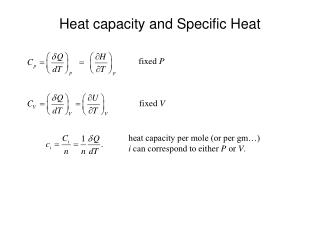DownloadDownload PresentationHeat capacity and Specific Heat

# Heat capacity and Specific Heat

Télécharger la présentation## Heat capacity and Specific Heat

- - - - - - - - - - - - - - - - - - - - - - - - - - - E N D - - - - - - - - - - - - - - - - - - - - - - - - - - -
##### Presentation Transcript

1. Heat capacity and Specific Heat fixed P fixed V heat capacity per mole (or per gm…) i can correspond to either P or V.

2. Heat capacity and Specific Heat Usually the for real substances specific heats are functions of temperature and we need to know ci(T) before we can evaluate Dh or Du.

3. large heat capacity small heat capacity Heat capacity and Specific Heat What physical processes control the heat capacity of a substance? Ideal Gas: Monatomic gas; 3 translational DOF. Each contributes kBT/2 to the internal energy.

4. Heat capacity and Specific Heat Monatomic Ideal Gas cont: Diatomic Ideal Gas: 3 translational and two rotational DOF. Each contributes kBT/2 to the internal energy. Note that the specific heat of an ideal gas does not change with temperature.

5. Heat capacity and Specific Heat What physical processes control the heat capacity of a substance? Crystalline solids 3 translational DOF + 3 vibrational DOF  3R Law of Dulong and Petit. Turns out that there is a temperature dependence to the specific heat . Low Temperatures Lattice vibrations ~ T 3 energy absorption by electrons ~ T K =1940 J/mol-K and g is material dependent

6. Heat capacity and Specific Heat TD Law of Dulong and Petit Also note that at very low T

7. Heat capacity and Specific Heat High temperatures Specific heats at temperatures (~above room temperature) are usually expressed by an empirical equation of the form: Here, a, b and c are material dependent constants. Note that since the equation is empirical the constants have no simple physical interpretation and that such equations should only be used over the temperature range for which the constants were experimentally determined.

8. Heat capacity and Specific Heat Relation between cp and cV for an ideal gas Statement of the 1st Law Ideal gas law (n = 1) Total derivative

9. Heat capacity and Specific Heat Recall the definitions of heat capacity In terms of the specific internal energy u and enthalpy h the heat capacities per unit mass are called specific heats and take on the following forms: The specific heat ratio, k, is a quantity that later we will find useful and is defined by,

10. Heat capacity and Specific Heat Approximations for Condensed Phases It turns out that the specific volume and specific internal energy of condensed phases vary little with pressure, In the case of liquids the specific volume and internal energy may be evaluated for engineering purposes at the condensed liquid state at the temperature of interest. This is the incompressible substance model. It’s an approximation.

11. Heat capacity and Specific Heat A similar approximation can be made for the specific enthalpy. Since, h = u + pv,

12. Heat capacity and Specific Heat In the incompressible substance approximation since the specific internal energy and volume are taken as functions of temperature only, Note that this is an ordinary derivative since u is only a function of T in this approximation. Also in this approximation, since taking derivatives while holding p fixed yields, This says that in this model,

13. Heat capacity and Specific Heat Then the changes in specific internal energy and enthalpy can be calculated according to: incompressible substance

14. The Ideal Gas Model The equation of state of an ideal gas is given by where P is the pressure, V the volume, R the gas constant, n the number of moles and T the absolute temperature (K). The gas constant R = 8.314 J/mole-K. There are various forms of this equation that you should become familiar with. Some text books are a little confusing on this issue. For example they define a symbol R different from the customary definition where the value of R is a constant for a particular gas of molecular weight M. Then the units of this R is kJ/kg-K. This is ugly since here R varies from material to material since the molecular weight is different for different materials. That is, R = R/M. This is ugly! It’s best just to know how to do the conversions. For example,

15. The Ideal Gas Model Also note that since R = 8.314 J/mole-K and 1 mole of a substance contains 6.02 x 1023 molecules (Avogadro’s number, NA), R/NA= 1.38 x 10-23J/molecule ºK. = kB. kB is known as Boltzmann’s constant. So, we can rewrite the ideal gas law as N is the no. of molecules

16. The Ideal Gas Model Now, there’s a real interesting question to ask. How does the internal energy, or enthalpy of an ideal gas depend on the state of the gas, i.e., the P, V, T values? Do you remember how an ideal gas is defined? The molecules do not interact with one another. The ideal gas equation connects the 3 variables, so it’s not possible to hold two of them constant and alter the 3rd to examine this problem. It turns out that the internal energy of an ideal gas only depends on temperature.

17. The First Law and the Ideal Gas Model du = q - w = q - pdv For the ideal gas u = u(T) so, and, (a) and substitution Also for 1 mole of an ideal gas, pv = RT, for pdv in Equation (a), (b) In a quasi-static adiabatic process since, q = 0,

18. The First Law and the Ideal Gas Model Dividing the first equation by the second and rearranging, Integration yields,